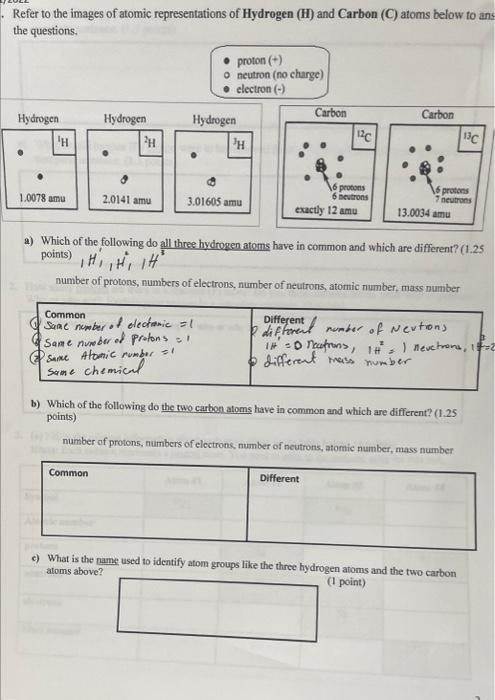# (Solved): please answer the following questions  Refer to the images of atomic representations of Hydroge ...Refer to the images of atomic representations of Hydrogen $$(\mathbf{H})$$ and Carbon $$(\mathbf{C})$$ atoms below to ans the questions. a) Which of the following do all three hydrogen atoms have in common and which are different? (1.25 points) , $$H_{1}^{\prime}, H_{1}^{2}, H^{3}$$ number of protons, numbers of electrons, number of neutrons, atomic number, mass number b) Which of the following do the two carben atoms have in common and which are different? $$(1.25$$ points) number of protons, numbers of electrons, number of neutrons, atomic number, mass number c) What is the name used to identify atom groups like the three hydrogen atoms and the two carbon atoms above? (1 point)

We have an Answer from Expert